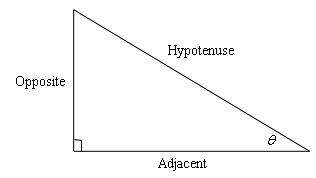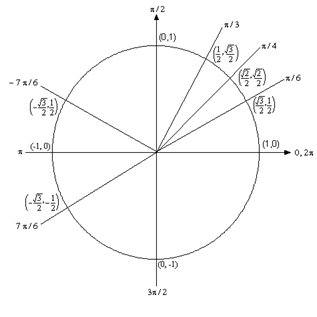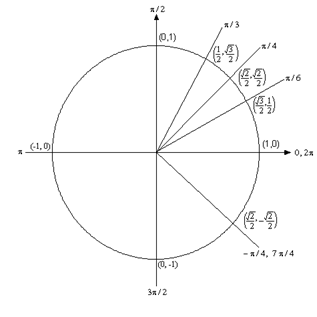Paul's Online Notes
Home / Calculus I / Review / Trig Functions
Show Mobile Notice Show All Notes Hide All Notes
Mobile Notice
You appear to be on a device with a "narrow" screen width (i.e. you are probably on a mobile phone). Due to the nature of the mathematics on this site it is best views in landscape mode. If your device is not in landscape mode many of the equations will run off the side of your device (should be able to scroll to see them) and some of the menu items will be cut off due to the narrow screen width.

### Section 1.3 : Trig Functions

The intent of this section is to remind you of some of the more important (from a Calculus standpoint…) topics from a trig class. One of the most important (but not the first) of these topics will be how to use the unit circle. We will leave the most important topic to the next section.

First let’s start with the six trig functions and how they relate to each other.

$\begin{array}{ll}{\cos \left( x \right)}&\hspace{0.75in}\sin \left( x \right)\\{\tan \left( x \right) = \displaystyle \frac{{\sin \left( x \right)}}{{\cos \left( x \right)}}}&\hspace{0.75in}\cot \left( x \right) = \displaystyle \frac{{\cos \left( x \right)}}{{\sin \left( x \right)}} = \displaystyle \frac{1}{{\tan \left( x \right)}}\\{\sec \left( x \right) = \displaystyle \frac{1}{{\cos \left( x \right)}}}&\hspace{0.75in}\csc \left( x \right) = \displaystyle \frac{1}{{\sin \left( x \right)}}\end{array}$

Recall as well that all the trig functions can be defined in terms of a right triangle.From this right triangle we get the following definitions of the six trig functions.

 $$\displaystyle \cos \theta = \frac{{{\rm{adjacent}}}}{{{\rm{hypotenuse}}}}$$ $$\displaystyle \sin \theta = \frac{{{\rm{opposite}}}}{{{\rm{hypotenuse}}}}$$ $$\displaystyle \tan \theta = \frac{{{\rm{opposite}}}}{{{\rm{adjacent}}}}$$ $$\displaystyle \cot \theta = \frac{{{\rm{adjacent}}}}{{{\rm{opposite}}}}$$ $$\displaystyle \sec \theta = \frac{{{\rm{hypotenuse}}}}{{{\rm{adjacent}}}}$$ $$\displaystyle \csc \theta = \frac{{{\rm{hypotenuse}}}}{{{\rm{opposite}}}}$$

Remembering both the relationship between all six of the trig functions and their right triangle definitions will be useful in this course on occasion.

Next, we need to touch on radians. In most trig classes instructors tend to concentrate on doing everything in terms of degrees (probably because it’s easier to visualize degrees). The same is true in many science classes. However, in a calculus course almost everything is done in radians. The following table gives some of the basic angles in both degrees and radians.

 Degree 0 30 45 60 90 180 270 360 Radians 0 $$\displaystyle \frac{\pi }{6}$$ $$\displaystyle \frac{\pi }{4}$$ $$\displaystyle \frac{\pi }{3}$$ $$\displaystyle \frac{\pi }{2}$$ $$\pi$$ $$\displaystyle \frac{{3\pi }}{2}$$ $$2\pi$$

Know this table! We may not see these specific angles all that much when we get into the Calculus portion of these notes, but knowing these can help us to visualize each angle. Now, one more time just make sure this is clear.

Be forewarned, everything in most calculus classes will be done in radians!

Let’s next take a look at one of the most overlooked ideas from a trig class. The unit circle is one of the more useful tools to come out of a trig class. Unfortunately, most people don’t learn it as well as they should in their trig class.

Below is unit circle with just the first quadrant filled in with the “standard” angles. The way the unit circle works is to draw a line from the center of the circle outwards corresponding to a given angle. Then look at the coordinates of the point where the line and the circle intersect. The first coordinate, i.e. the $$x$$-coordinate, is the cosine of that angle and the second coordinate, i.e. the $$y$$-coordinate, is the sine of that angle. We’ve put some of the standard angles along with the coordinates of their intersections on the unit circle.So, from the unit circle above we can see that $$\cos \left( {\frac{\pi }{6}} \right) = \frac{{\sqrt 3 }}{2}$$ and $$\sin \left( {\frac{\pi }{6}} \right) = \frac{1}{2}$$.

Also, remember how the signs of angles work. If you rotate in a counter clockwise direction the angle is positive and if you rotate in a clockwise direction the angle is negative.

Recall as well that one complete revolution is $$2\pi$$, so the positive $$x$$-axis can correspond to either an angle of 0 or $$2\pi$$ (or $$4\pi$$, or $$6\pi$$, or $$- 2\pi$$, or $$- 4\pi$$, etc. depending on the direction of rotation). Likewise, the angle $${\textstyle{\pi \over 6}}$$ (to pick an angle completely at random) can also be any of the following angles:

$$\displaystyle \frac{\pi }{6} + 2\pi = \frac{{13\pi }}{6}$$ (start at $$\displaystyle \frac{\pi }{6}$$ then rotate once around counter clockwise)

$$\displaystyle \frac{\pi }{6} + 4\pi = \frac{{25\pi }}{6}$$ (start at $$\displaystyle \frac{\pi }{6}$$ then rotate around twice counter clockwise)

$$\displaystyle \frac{\pi }{6} - 2\pi = - \frac{{11\pi }}{6}$$ (start at $$\displaystyle \frac{\pi }{6}$$ then rotate once around clockwise)

$$\displaystyle \frac{\pi }{6} - 4\pi = - \frac{{23\pi }}{6}$$ (start at $$\displaystyle \frac{\pi }{6}$$ then rotate around twice clockwise)

etc.

In fact, $${\textstyle{\pi \over 6}}$$ can be any of the following angles $${\frac{\pi}{6}} + 2\pi \,n\,,\;\;n = 0,\, \pm 1,\, \pm 2,\, \pm 3,\, \ldots$$ In this case $$n$$ is the number of complete revolutions you make around the unit circle starting at $${\frac{\pi}{6}}$$. Positive values of $$n$$ correspond to counter clockwise rotations and negative values of $$n$$ correspond to clockwise rotations.

So, why did we only put in the first quadrant? The answer is simple. If you know the first quadrant then you can get all the other quadrants from the first with a small application of geometry. You’ll see how this is done in the following set of examples.

Example 1 Evaluate each of the following.
1. $$\sin \left( {\frac{{2\pi }}{3}} \right)$$ and $$\sin \left( { - \frac{{2\pi }}{3}} \right)$$
2. $$\cos \left( {\frac{{7\pi }}{6}} \right)$$ and $$\cos \left( { - \frac{{7\pi }}{6}} \right)$$
3. $$\tan \left( { - \frac{\pi }{4}} \right)$$ and $$\tan \left( {\frac{{7\pi }}{4}} \right)$$
4. $$\sec \left( {\frac{{25\pi }}{6}} \right)$$
Show All Solutions Hide All Solutions
a $$\sin \left( {\frac{{2\pi }}{3}} \right)$$ and $$\sin \left( { - \frac{{2\pi }}{3}} \right)$$ Show Solution

The first evaluation in this part uses the angle $$\frac{{2\pi }}{3}$$. That’s not on our unit circle above, however notice that $$\frac{{2\pi }}{3} = \pi - \frac{\pi }{3}$$. So $$\frac{{2\pi }}{3}$$ is found by rotating up $$\frac{\pi }{3}$$ from the negative $$x$$-axis. This means that the line for $$\frac{{2\pi }}{3}$$ will be a mirror image of the line for $$\frac{\pi }{3}$$ only in the second quadrant. The coordinates for $$\frac{{2\pi }}{3}$$ will be the coordinates for $$\frac{\pi }{3}$$ except the $$x$$ coordinate will be negative.

Likewise, for $$- \frac{{2\pi }}{3}$$we can notice that $$- \frac{{2\pi }}{3} = - \pi + \frac{\pi }{3}$$, so this angle can be found by rotating down $$\frac{\pi }{3}$$ from the negative $$x$$-axis. This means that the line for $$- \frac{{2\pi }}{3}$$ will be a mirror image of the line for $$\frac{\pi }{3}$$ only in the third quadrant and the coordinates will be the same as the coordinates for $$\frac{\pi }{3}$$ except both will be negative.

Both of these angles, along with the coordinates of the intersection points, are shown on the following unit circle.From this unit circle we can see that $$\sin \left( {\frac{{2\pi }}{3}} \right) = \frac{{\sqrt 3 }}{2}$$and $$\sin \left( { - \frac{{2\pi }}{3}} \right) = - \frac{{\sqrt 3 }}{2}$$.

This leads to a nice fact about the sine function. The sine function is called an odd function and so for ANY angle we have

$\sin \left( { - \theta } \right) = - \sin \left( \theta \right)$

b $$\cos \left( {\frac{{7\pi }}{6}} \right)$$ and $$\cos \left( { - \frac{{7\pi }}{6}} \right)$$ Show Solution

For this example, notice that $$\frac{{7\pi }}{6} = \pi + \frac{\pi }{6}$$ so this means we would rotate down $$\frac{\pi }{6}$$ from the negative $$x$$-axis to get to this angle. Also $$- \frac{{7\pi }}{6} = - \pi - \frac{\pi }{6}$$ so this means we would rotate up $$\frac{\pi }{6}$$ from the negative $$x$$-axis to get to this angle. So, as with the last part, both of these angles will be mirror images of $$\frac{\pi }{6}$$ in the third and second quadrants respectively and we can use this to determine the coordinates for both of these new angles.

Both of these angles are shown on the following unit circle along with the coordinates for the intersection points.From this unit circle we can see that $$\cos \left( {\frac{{7\pi }}{6}} \right) = - \frac{{\sqrt 3 }}{2}$$and $$\cos \left( { - \frac{{7\pi }}{6}} \right) = - \frac{{\sqrt 3 }}{2}$$. In this case the cosine function is called an even function and so for ANY angle we have

$\cos \left( { - \theta } \right) = \cos \left( \theta \right)$

c $$\tan \left( { - \frac{\pi }{4}} \right)$$ and $$\tan \left( {\frac{{7\pi }}{4}} \right)$$ Show Solution

Here we should note that $$\frac{{7\pi }}{4} = 2\pi - \frac{\pi }{4}$$ so $$\frac{{7\pi }}{4}$$ and $$- \frac{\pi }{4}$$ are in fact the same angle! Also note that this angle will be the mirror image of $$\frac{\pi }{4}$$ in the fourth quadrant. The unit circle for this angle isNow, if we remember that $$\tan \left( x \right) = \frac{{\sin \left( x \right)}}{{\cos \left( x \right)}}$$ we can use the unit circle to find the values of the tangent function. So,

$\tan \left( \frac{7\pi }{4} \right)=\tan \left( -\frac{\pi }{4} \right)=\frac{\sin \left( -{\pi }/{4}\; \right)}{\cos \left( -{\pi }/{4}\; \right)}=\frac{-{\sqrt{2}}/{2}\;}{{\sqrt{2}}/{2}\;}=-1$

On a side note, notice that $$\tan \left( {\frac{\pi }{4}} \right) = 1$$ and we can see that the tangent function is also called an odd function and so for ANY angle we will have

$\tan \left( { - \theta } \right) = - \tan \left( \theta \right)$

d $$\sec \left( {\frac{{25\pi }}{6}} \right)$$ Show Solution

Here we need to notice that $$\frac{{25\pi }}{6} = 4\pi + \frac{\pi }{6}$$. In other words, we’ve started at $$\frac{\pi }{6}$$ and rotated around twice to end back up at the same point on the unit circle. This means that

$\sec \left( {\frac{{25\pi }}{6}} \right) = \sec \left( {4\pi + \frac{\pi }{6}} \right) = \sec \left( {\frac{\pi }{6}} \right)$

Now, let’s also not get excited about the secant here. Just recall that

$\sec \left( x \right) = \frac{1}{{\cos \left( x \right)}}$

and so all we need to do here is evaluate a cosine! Therefore,

$\sec \left( \frac{25\pi }{6} \right)=\sec \left( \frac{\pi }{6} \right)=\frac{1}{\cos \left( \frac{\pi }{6} \right)}=\frac{1}{{}^{\sqrt{3}}/{}_{2}}=\frac{2}{\sqrt{3}}$

So, in the last example we saw how the unit circle can be used to determine the value of the trig functions at any of the “common” angles. It’s important to notice that all of these examples used the fact that if you know the first quadrant of the unit circle and can relate all the other angles to “mirror images” of one of the first quadrant angles you don’t really need to know whole unit circle. If you’d like to see a complete unit circle I’ve got one on my Trig Cheat Sheet that is available at http://tutorial.math.lamar.edu.

Another important idea from the last example is that when it comes to evaluating trig functions all that you really need to know is how to evaluate sine and cosine. The other four trig functions are defined in terms of these two so if you know how to evaluate sine and cosine you can also evaluate the remaining four trig functions.

We’ve not covered many of the topics from a trig class in this section, but we did cover some of the more important ones from a calculus standpoint. There are many important trig formulas that you will use occasionally in a calculus class. Most notably are the half-angle and double-angle formulas. If you need reminded of what these are, you might want to download my Trig Cheat Sheet as most of the important facts and formulas from a trig class are listed there.